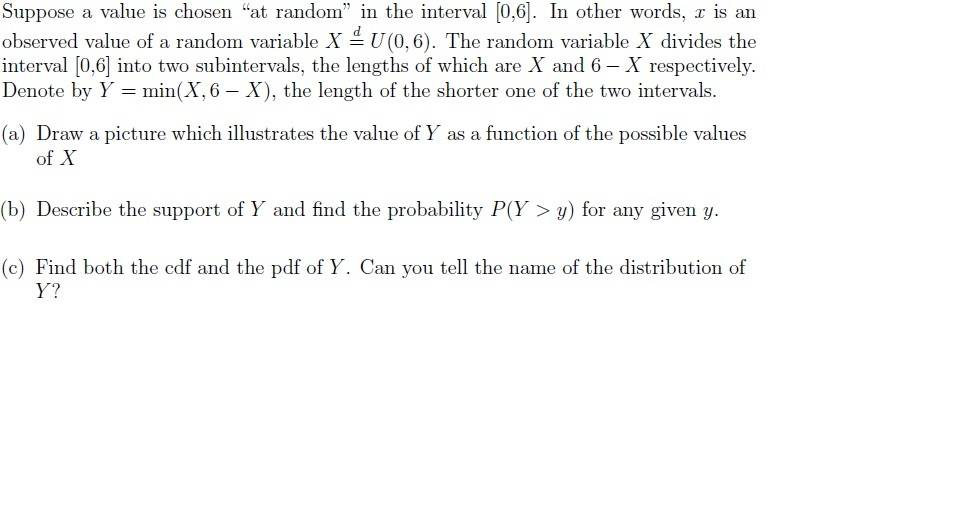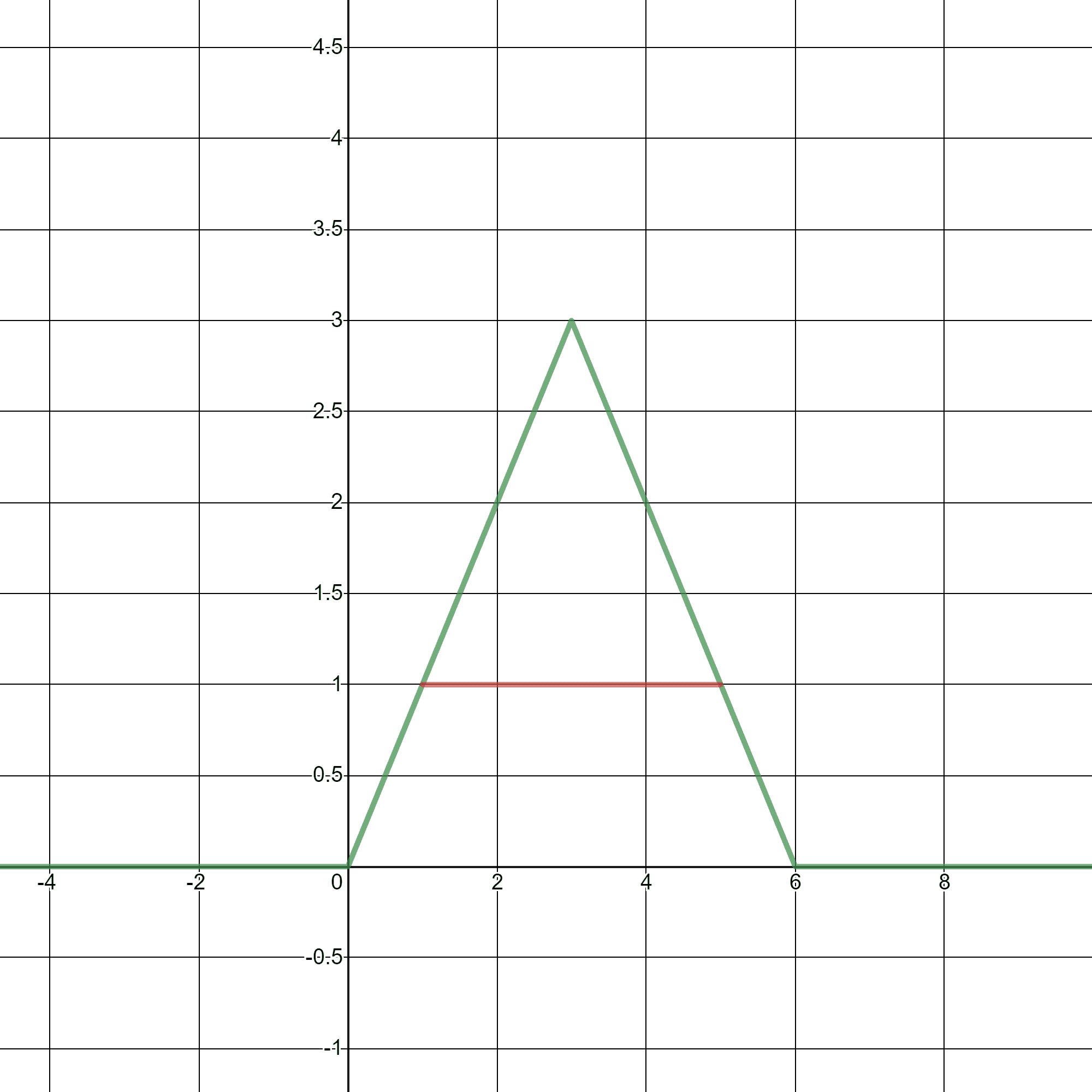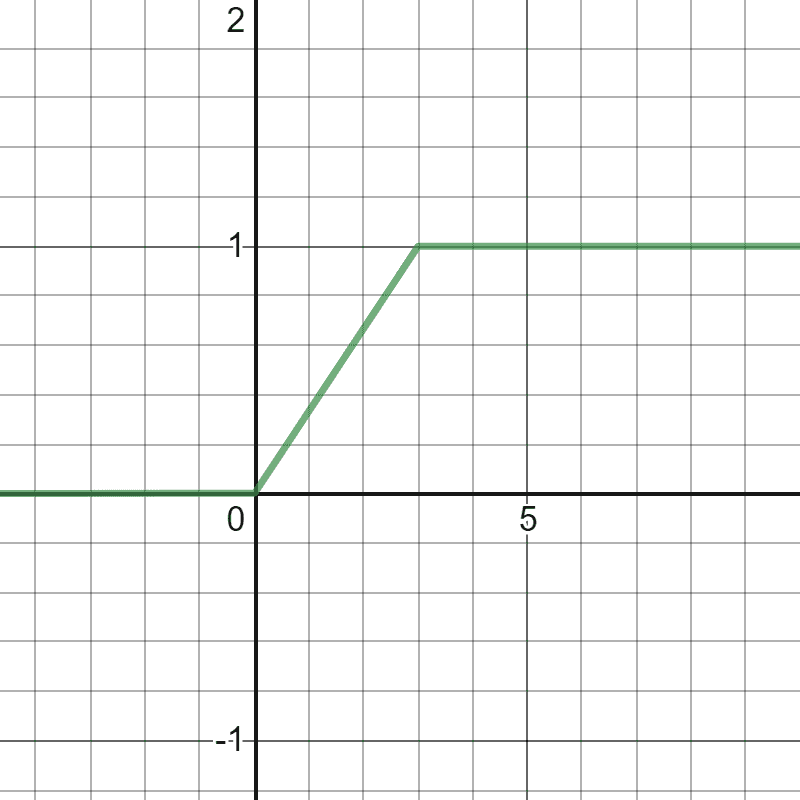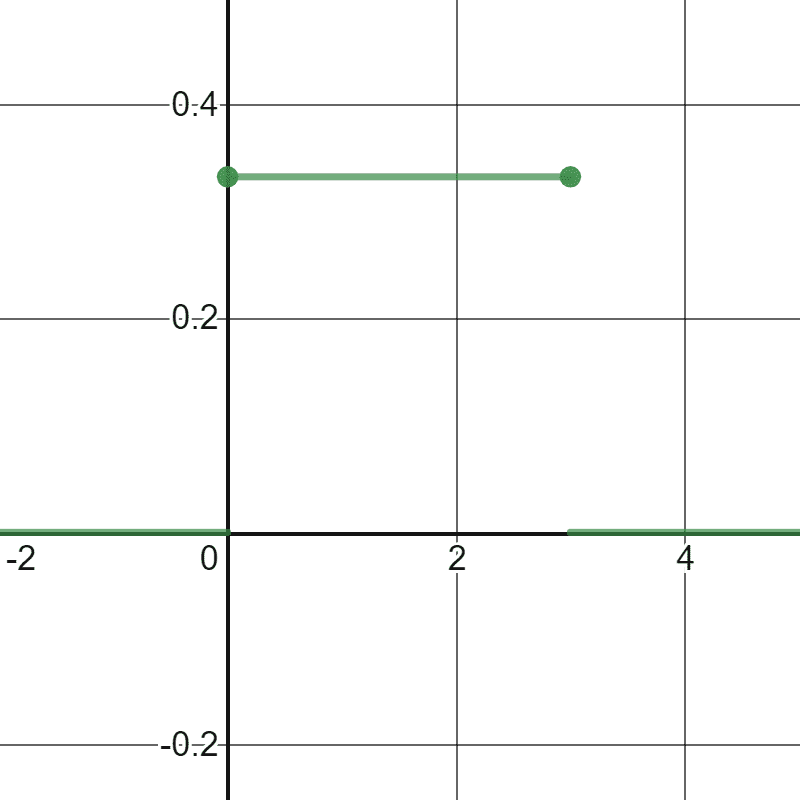# Uniform distribution question

• MHB
• lemonthree

#### lemonthree

I need guidance on part c, finding the cdf/pdf of Y.

I understand that for X>3, Y=6-X and for X<3, Y=X.
For X = 3, Y=3

For part b, I got P(Y>y)= (3-y)/3, for 0≤y<3

Now for part c, I know P(Y>y) relates to the cdf.
But the definition of cdf relates to P(Y<y), so I'm guessing I have to
do 1-P(Y>y) to get the cdf which is y/3, 0≤y<3.

I'm thinking the pdf would be 1/3 for 0≤y<3?

I know for sure Y is a uniform distribution.
I'm not too sure on the interval (x-a)/(b-a)
Is it Y~Uniform distribution(0,3)?Hi lemonthree,

The short answer to your post is that you're basically correct on everything you wrote. This response is really to help clean up some of the details. Please refer to the image below, which is a plot of the random variable $Y$ as a function of $X$, as defined by $Y = \min\{X, 6-X\}$.

For completeness in part (b), the values for $P(Y>y)$ should be included for $y\leq 0$ and $y\geq 3$. From the graph below we see that

$P(Y > y) = \begin{cases} 1 & y\leq 0\\ 1-\frac{y}{3} & 0\leq y\leq 3\\ 0 & y\geq 3 \end{cases}$

For part (c) you can certainly use $P(Y<y) = 1-P(Y>y)$ and the formula from part (b) to get

$P(Y < y) = \begin{cases} 0 & y\leq 0\\ \frac{y}{3} & 0\leq y\leq 3\\ 1 & y\geq 3 \end{cases}$

Another possibility is to reason geometrically. For example, if $y = 1$ (as shown in the figure), we can see that $P(Y < 1) = P(X<1\,\text{or}\, X>5) = 2\cdot P(X <1) = 1/3,$ where the second equality is true because the graph is symmetric about the vertical line $X = 3.$ This same argument can be used for any $y$ such that $0\leq y\leq 3$; i.e., $P(Y<y) = P(X<y\,\text{or}\, X>6-y) = 2\cdot P(X<y) = \frac{2y}{6}=\frac{y}{3}$ for all $0\leq y\leq 3$.The CDF is pictured below. The PDF is obtained by taking the derivative of the CDF. I suspect you're familiar with derivatives. If not, derivative means the slope of a graph. Hence, when we say the PDF is the derivative of the CDF, we mean we get the PDF by looking at the slopes of the CDF. Because there are sharp corners in the graph of the CDF, it is technically not differentiable at these points, which results in so-called "jump discontinuities" in the PDF. In any case, the resulting PDF is given by

$f(t) = \begin{cases} 0 & t< 0\\ \frac{1}{3} & 0\leq t\leq 3\\ 0 & t> 3 \end{cases}$Last edited:
Thank you so much GJA. That was very helpful. I just have a question on this part regarding the inequality signs.

For completeness in part (b), the values for $P(Y>y)$ should be included for $y\leq 0$ and $y\geq 3$. From the graph below we see that

$P(Y > y) = \begin{cases} 1 & y\leq 0\\ 1-\frac{y}{3} & 0\leq y\leq 3\\ 0 & y\geq 3 \end{cases}$

Should it be
$P(Y > y) = \begin{cases} 1 & y\ < 0\\ 1-\frac{y}{3} & 0\leq y\leq 3\\ 0 & y\ > 3 \end{cases}$
instead? Or both ways work fine? Although the values are the same I'm not very sure whether I'm mathematically correct...

Since the values are the same, both answers are acceptable. Because the values are the same, the CDF is a "continuous" function; meaning there aren't jumps/different values at the points $y=0$ and $y=3$. For comparison, the PDF is not continuous at $y=0$ and $y=3$. This can be seen as jumps in the graph below. For the PDF, you can see that we used strict inequalities to define $f(t)$.